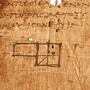1. Forum
2. >
3. Topic: German
4. >I don't see a need for the mixed declension table. I know its supposed to be for adjectives preceded by -ein words (ein, kein, mein, irgendein, sein etc) but in those instances I just use the strong table when the -ein word has no ending and the weak table when the -ein word does have an ending.

Eg: Mein alter Freund schreibt mit seinem neuen Bleistift.

"mein" is endingless so use the strong table ending (-er for masculine nominative) and "seinem" has an ending so use weak declension ending (-en for masculine dative)

So is the mixed declension table totally redundant?.

Also, to simplify things further, if you learn the definite article table then you can derive the strong adjective endings table from that as follows : der(-er), die(-e), das(-es), den(-en), dem(-em) and des(-en - NOT -es)

Eg: Ich glaube, kaltes Bier schmeckt gut.

Bier is nominative neuter (das Bier) so the ending is -es, kaltes.

So, you may only need to learn the weak declension table which is nearly all -en apart from the five -e endings.

Unless I have missed something (let me know if i have), this approach seems to work and I find it much easier than the three table approach.

April 8, 2018

## 1 CommentI agree that using tables is a terrible way to teach this, and yet somehow it's what most textbooks and courses do. If you already know when to use the different forms of 'der' and 'ein' then the whole thing can be reduced to a few simple rules. This can be done in different ways though so there is some choice in how you want to formulate these rules. Here is my version:

If there is no article then go according to the form of 'der' you would use. Otherwise if it's plural, or if it's Dative or Genitive case then add -en. Otherwise if there's an indefinite article the go according to the form of 'der' and if there is an definite article then go according to the form of 'ein'.

The Strong/Weak/Mixed just adds an extra layer of jargon without really making the system any easier.